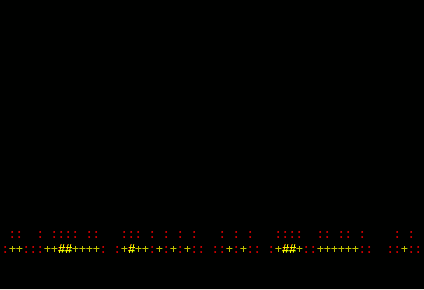Published: 20. 12. 2010   Category: GNU/Linux

# BASH on fire

A question that worries shell programmers for ages was answered! How to make some classic demo effect like fire in Bourne again shell? :-) See the answer below.## Updated version (2012-JAN-17)

I was playing with the code, to made some updates. First of all, I test size of terminal with tput command. Also there are few minor changes to clean up the code, so I saved almost 100 bytes! I've tried to improve the speed, but any change is not noticeable :(

The fire effect is computed inside one big string (columns × rows) and the numbers inside the string addressing pixel palette \$B. You can display this palette with:

```B=(' ' '\E[0;31m.' '\E[0;31m:' '\E[1;31m+' '\E[0;33m+' '\E[1;33mU' '\E[1;33mW');
echo -e \${B[*]}
```

Each „pixel“ contains ANSI code for terminal color and some character to create the fading effect.

The nice description of fire algorithm can be find here.

```#!/bin/bash
X=`tput cols` Y=`tput lines` e=echo M=`eval \$e {1..\$[X*Y]}` P=`eval \$e {1..\$X}`;
B=(' ' '\E[0;31m.' '\E[0;31m:' '\E[1;31m+' '\E[0;33m+' '\E[1;33mU' '\E[1;33mW');
\$e -e "\E[2J\E[?25l" ; while true; do p=''; for j in  \$P; do p=\$p\$[\$RANDOM%2*9];
done;O=\${C:0:\$[X*(Y-1)]}\$p;C='' S='';for p in \$M;do #  _-=[ BruXy.RegNet.CZ ]=-_
read a b c d <<< "\${O:\$[p+X-1]:1} \${O:\$[p+X]:1} \${O:\$[p+X+1]:1} \${O:\$[p+X+X]:1}"
v=\$[(a+b+c+d)/4] C=\$C\$v S=\$S\${B[\$v]}; done; printf "\E[1;1f\$S"; done  # (c) 2012
```

## Old version

Copy and save the following code, make it executable using chmod +x fire.sh and run it with parametr ./fire.sh \$COLUMNS. This variable contains number of columns in current terminal window. I recommend to change the window size to see the effect in better speed.

``` #!/bin/bash e=echo\ -e X=\$1 Y=\$[(X*5)/16] M=`eval \$e {1..\$[X*Y]}` ####### P=`eval \$e {1..\$X}}`;for i in \$M; do S=\$S"0";done;u=0; ###### B=(" " "\E[0;31m." "\E[0;31m:" "\E[1;31m+" "\E[0;33m+" ##### "\E[1;33mU" "\E[1;33mW");\$e "\E[2J\E[?25l";while true;do #### p='';for j in \$P;do p=\$p\$[\$RANDOM%2*9];done; [ \$u -gt 0 ] \ &&O=\${C:0:\$[X*(Y-1)]}\$p; [ \$[u++] -eq 0 ] && O=\$S\$p;C='' #### S=''; for p in \$M; do a=\${O:\$[p+X-1]:1} b=\${O:\$[p+X]:1} ##### c=\${O:\$[p+X+1]:1} d=\${O:\$[p+X+X]:1} v=\$[ (a+b+c+d)/4] ###### C=\$C\$v S=\$S\${B[\$v]}; done; \$e "\E[1;1f";\$e "\$S";done #BruXy# ```